# Drop Sum

Drop Sum is an 8-bit style math game for kids to practice addition. Drop Sum has 2 modes of play: Make 10 & Random. In Make 10 mode kids must add falling blocks together to equal 10. In Random mode kids must add falling blocks together to equal a range of numbers 2-10. The goal of Drop Sum is to collect as many coins as possible by correctly adding together blocks to equal the target number. The game is over when a stack of blocks reaches the top of the screen. TIP: Stacks of blocks greater than ten will become frozen and stuck!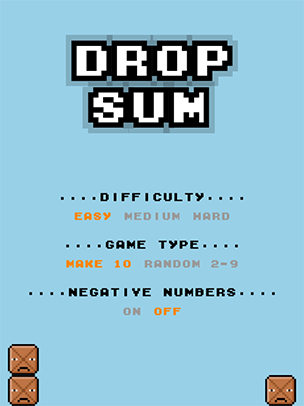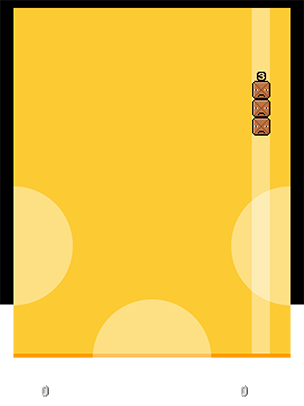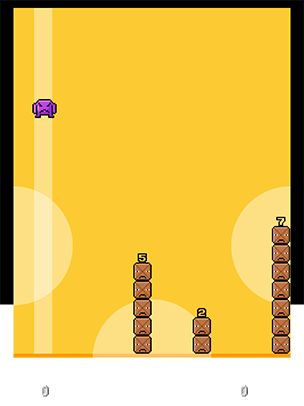Drop Sum is an 8-bit style math game for kids to practice addition. Drop Sum has 2 modes of play: Make 10 & Random. In Make 10 mode kids must add falling blocks together to equal 10. In Random mode kids must add falling blocks together to equal a range of numbers 2-10. The goal of Drop Sum is to collect as many coins as possible by correctly adding together blocks to equal the target number. The game is over when a stack of blocks reaches the top of the screen. TIP: Stacks of blocks greater than ten will become frozen and stuck!

# Drop Sum

Drop Sum is an 8-bit style math game for kids to practice addition. Drop Sum has 2 modes of play: Make 10 & Random. In Make 10 mode kids must add falling blocks together to equal 10. In Random mode kids must add falling blocks together to equal a range of numbers 2-10. The goal of Drop Sum is to collect as many coins as possible by correctly adding together blocks to equal the target number. The game is over when a stack of blocks reaches the top of the screen. TIP: Stacks of blocks greater than ten will become frozen and stuck!

Drop Sum is an 8-bit style math game for kids to practice addition. Drop Sum has 2 modes of play: Make 10 & Random. In Make 10 mode kids must add falling blocks together to equal 10. In Random mode kids must add falling blocks together to equal a range of numbers 2-10. The goal of Drop Sum is to collect as many coins as possible by correctly adding together blocks to equal the target number. The game is over when a stack of blocks reaches the top of the screen. TIP: Stacks of blocks greater than ten will become frozen and stuck!Drop Sum is an 8-bit style math game for kids to practice addition. Drop Sum has 2 modes of play: Make 10 & Random. In Make 10 mode kids must add falling blocks together to equal 10. In Random mode kids must add falling blocks together to equal a range of numbers 2-10. The goal of Drop Sum is to collect as many coins as possible by correctly adding together blocks to equal the target number. The game is over when a stack of blocks reaches the top of the screen. TIP: Stacks of blocks greater than ten will become frozen and stuck!Drop Sum is an 8-bit style math game for kids to practice addition. Drop Sum has 2 modes of play: Make 10 & Random. In Make 10 mode kids must add falling blocks together to equal 10. In Random mode kids must add falling blocks together to equal a range of numbers 2-10. The goal of Drop Sum is to collect as many coins as possible by correctly adding together blocks to equal the target number. The game is over when a stack of blocks reaches the top of the screen. TIP: Stacks of blocks greater than ten will become frozen and stuck!

# Drop Sum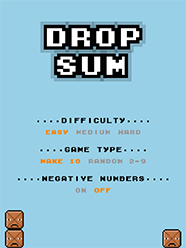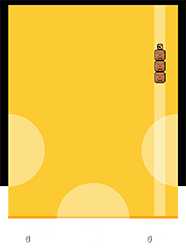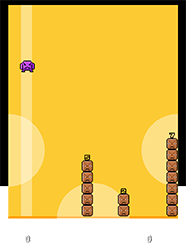Drop Sum is an 8-bit style math game for kids to practice addition. Drop Sum has 2 modes of play: Make 10 & Random. In Make 10 mode kids must add falling blocks together to equal 10. In Random mode kids must add falling blocks together to equal a range of numbers 2-10. The goal of Drop Sum is to collect as many coins as possible by correctly adding together blocks to equal the target number. The game is over when a stack of blocks reaches the top of the screen. TIP: Stacks of blocks greater than ten will become frozen and stuck!